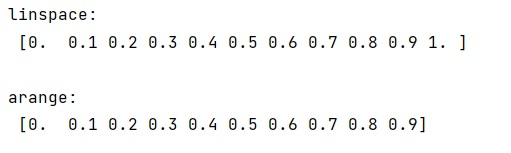# What is the difference between np.linspace() and np.arange() methods?

Learn about the difference between np.linspace() and np.arange() methods in Python?
Submitted by Pranit Sharma, on February 14, 2023

NumPy is an abbreviated form of Numerical Python. It is used for different types of scientific operations in python. Numpy is a vast library in python which is used for almost every kind of scientific or mathematical operation. It is itself an array which is a collection of various methods and functions for processing the arrays.

## numpy.linspace() Vs numpy.arange()

The numpy.arange() returns evenly spaced values within a given interval. Values are generated within the half-open interval [start, stop) (in other words, the interval includes the start but excludes the stop). For integer arguments, the function is equivalent to the Python built-in range function but returns a ndarray rather than a list.

The numpy.linspace() returns evenly spaced numbers over a specified interval. The output is num; evenly spaced samples, calculated over the interval [start, stop]. The endpoint of the interval can optionally be excluded.

The numpy.linspace() allows us to define how many values we get including the specified min and max values.

The numpy.arange() allows us to define the stepsize and infers the number of steps(the number of values we get).

Let us understand with the help of an example,

## Python code to demonstrate the example of np.linspace() vs np.arange() methods

```# Import numpy
import numpy as np

# Using linspace
print("linspace:\n",np.linspace(0,1,11),"\n")

# Using arange
print("arange:\n",np.arange(0,1,.1),"\n")
```

Output: